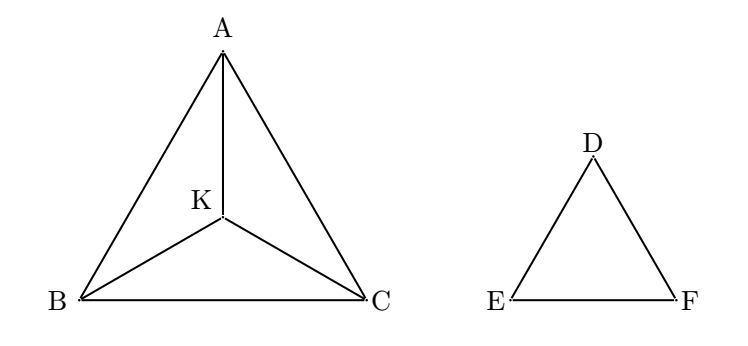# GrobyReplay of EWU Individual...
Limits 1s, 512 MB

In the 1970-80s, students in the Soviet Union had to face quite a hurdle. The administrators of selection exams of the Mathematics Department of Moscow State University, the most prestigious mathematics school in Russia, wanted to keep certain individuals from qualifying. Consequently, they came up with the idea of giving them selection exams that contained impossibly hard problems. Any student who failed to answer could be easily rejected, so this system was an effective method of controlling admissions. But these problems were not just difficult, they were carefully designed to have extremely simple solution. Yes, quite the contradiction! But bear with me. As you can imagine, it was not uncommon for students to protest and accuse the administrators of giving impossibly hard problems. In order to silence these protests, the administrators made sure that once the solutions were presented to them, they would seem foolishly simple. But of course while the solutions were simple, the journey to those said solutions were anything but. These problems were known as Coffins, which is a literal translation from the Russian word "Groby". Let's focus on one of such problems today.An equilateral triangle is a triangle in which all three sides are equal. Consider an equilateral triangle $ABC$. We pick any point $K$ inside the triangle and join $AK$, $BK$ and $CK$. Let us denote $\angle AKB$ as $x$ and $\angle BKC$ as $y$. Now we create a triangle using $AK$, $BK$ and $CK$. Let's call the triangle $\triangle DEF$, where $DE=AK$, $EF=BK$, $DF=CK$. Given $x$ and$y$, find out $\angle DEF$, $\angle DFE$ and $\angle EDF$.

## Input

The first line will contain a single integer $T$ ($1 \le T \le 10^5$) denoting the number of test cases.

The following $T$ lines will contain $x$ and $y$, separated by a single space. The angles will be valid.

## Output

For each test case, output the three angles in ascending order. Print a newline after each output. Error less than $10^{-6}$ will be ignored.

## Sample

InputOutput
1
120 120

60 60 60AdhocuDebug

### Submit